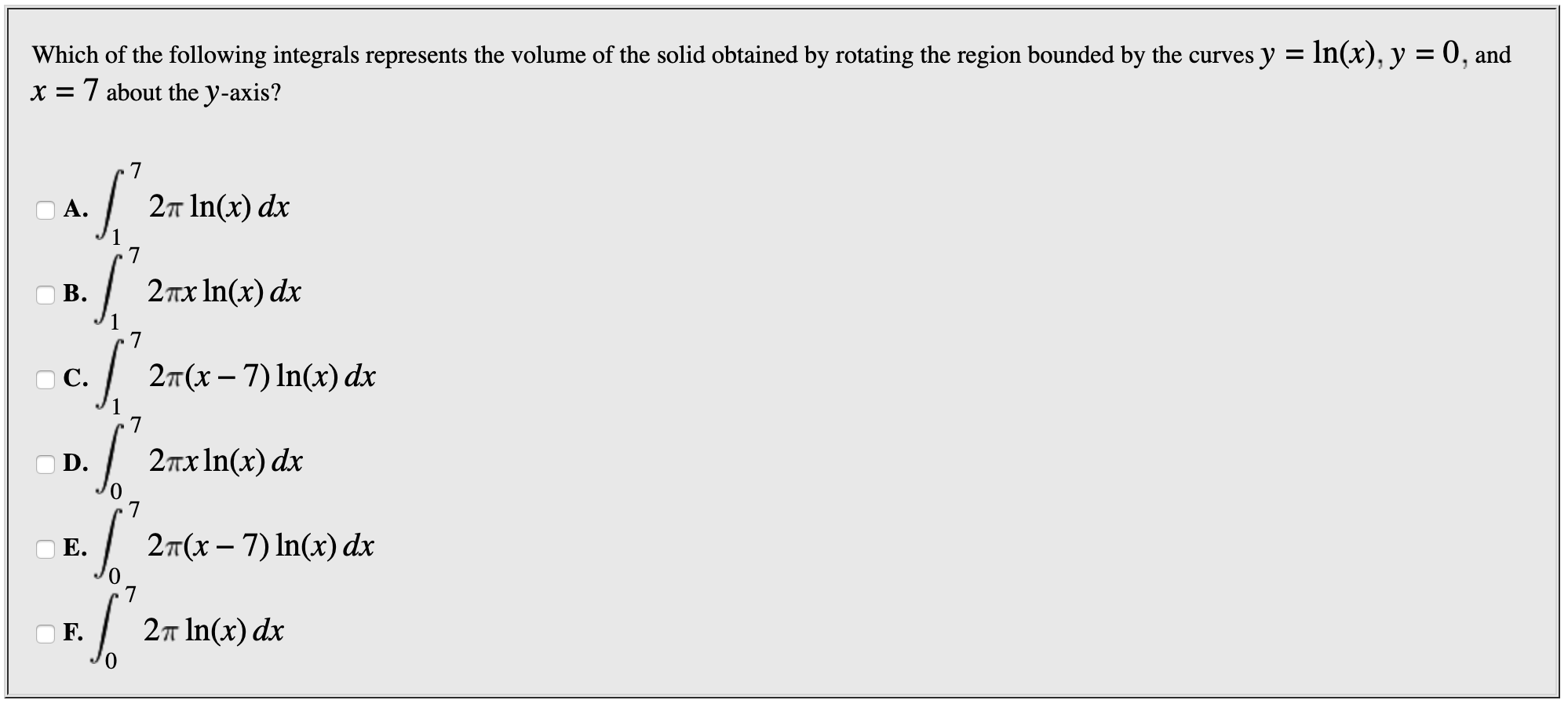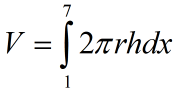# Which of the following integrals represents the volume of the solid obtained by rotating the region bounded by the curves y = In(x), y = 0, andx 7 about the y-axis?72т In(x) dxА.72тх In(x) dxВ.7c. 2T(x-7) In(x) dx72пх1n(х) dxD.. 2T(x-7) In(x) dx2п In(x) dxF.0

Question
6 views

problem in photo attachedhelp_outlineImage TranscriptioncloseWhich of the following integrals represents the volume of the solid obtained by rotating the region bounded by the curves y = In(x), y = 0, and x 7 about the y-axis? 7 2т In(x) dx А. 7 2тх In(x) dx В. 7 c. 2T(x-7) In(x) dx 7 2пх1n(х) dx D. . 2T(x-7) In(x) dx 2п In(x) dx F. 0 fullscreen
check_circle

Step 1

In this given curve points of intersection are,

Step 2

volume by a shell formula is given by the formula as,Step 3

in this curve 'r' and '...

### Want to see the full answer?

See Solution

#### Want to see this answer and more?

Solutions are written by subject experts who are available 24/7. Questions are typically answered within 1 hour.*

See Solution
*Response times may vary by subject and question.
Tagged in

### Integration